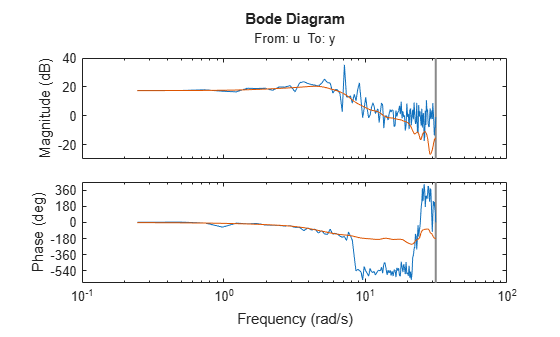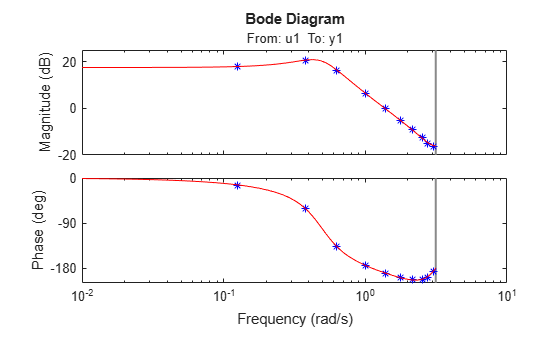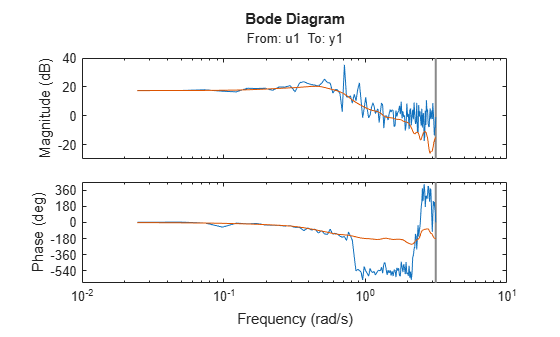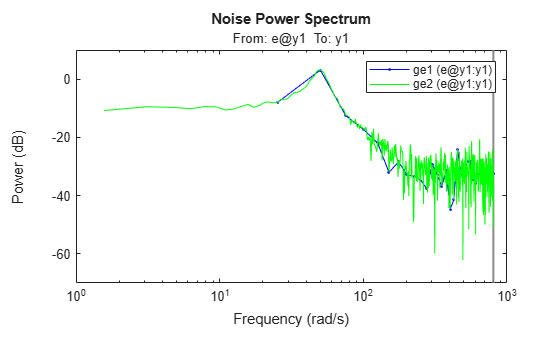# etfe

Estimate empirical transfer functions and periodograms

## Syntax

``g = etfe(data)``
``g = etfe(data,M)``
``g = etfe(data,M,N)``

## Description

example

````g = etfe(data)` estimates a transfer function of the form: $y\left(t\right)=G\left(q\right)u\left(t\right)+v\left(t\right)$`data` contains time- or frequency-domain input-output data or time-series data:If `data` is time-domain input-output signals, `g` is the ratio of the output Fourier transform to the input Fourier transform for the data. For nonperiodic data, the transfer function is estimated at 128 equally-spaced frequencies `[1:128]/128*pi/Ts`.For periodic data that contains a whole number of periods (```data.Period = integer``` ), the response is computed at the frequencies `k*2*pi/period` for ```k = 0``` up to the Nyquist frequency.If `data` is frequency-domain input-output signals, `g` is the ratio of output to input at all frequencies, where the input is nonzero.If `data` is time-series data (no input channels), `g` is the periodogram, that is the normed absolute square of the Fourier transform, of the data. The corresponding spectral estimate is normalized, as described in Spectrum Normalization and differs from the `spectrum` normalization in the Signal Processing Toolbox™ product.```

example

````g = etfe(data,M)` applies a smoothing operation on the raw spectral estimates using a Hamming Window that yields a frequency resolution of about `pi/M`. The effect of `M` is similar to the effect of `M` in `spa`. `M` is ignored for periodic data. Use this syntax as an alternative to `spa` for narrowband spectra and systems that require large values of `M`. ```

example

````g = etfe(data,M,N)` specifies the frequency spacing for nonperiodic data.For nonperiodic time-domain data, `N` specifies the frequency grid `[1:N]/N*pi/Ts` rad/TimeUnit. When not specified, `N` is 128.For periodic time-domain data, `N` is ignored.For frequency-domain data, the `N` is `fmin:delta_f:fmax`, where `[fmin fmax]` is the range of frequencies in `data`, and `delta_f` is `(fmax-fmin)/(N-1)` rad/TimeUnit. When not specified, the response is computed at the frequencies contained in data where input is nonzero.```

## Examples

collapse all

`load iddata1 z1;`

Estimate empirical transfer function and smoothed spectral estimate.

```ge = etfe(z1); gs = spa(z1);```

Compare the two models on a Bode plot.

`bode(ge,gs)`Generate a periodic input, simulate a system with it, and compare the frequency response of the estimated model with the original system at the excited frequency points.

Generate a periodic input signal and output signal using simulation.

```m = idpoly([1 -1.5 0.7],[0 1 0.5]); u = iddata([],idinput([50,1,10],'sine')); u.Period = 50; y = sim(m,u);```

Estimate an empirical transfer function.

`me = etfe([y u]);`

Compare the empirical transfer function with the original model.

`bode(me,'b*',m,'r')`Perform a smoothing operation on raw spectral estimates using a Hamming Window and compare the responses.

`load iddata1`

Estimate empirical transfer functions with and without the smoothing operation.

```ge1 = etfe(z1); ge2 = etfe(z1,32);```

Compare the models on a Bode plot.

`ge2` is smoother than `ge1` because of the effect of the smoothing operation.

`bode(ge1,ge2)`Estimate empirical transfer functions with low- and high-frequency spacings and compare the responses.

`load iddata9`

Estimate empirical transfer functions with low and high frequency spacings.

```ge1 = etfe(z9,[],32); ge2 = etfe(z9,[],512);```

Plot the output power spectrum of the two models.

`spectrum(ge1,'b.-',ge2,'g')`## Input Arguments

collapse all

Estimation data, specified as an `iddata` object. The data can be time- or frequency-domain input/output signals or time-series data.

Frequency resolution, specified as a positive scalar.

Frequency spacing, specified as a positive scalar. For frequency-domain data, the default frequency spacing is the spacing inherent in the estimation data.

## Output Arguments

collapse all

Transfer function estimate, returned as an `idfrd` model.

Information about the estimation results and options used is stored in the model's `Report` property. `Report` has the following fields:

Report FieldDescription
`Status`

Summary of the model status, which indicates whether the model was created by construction or obtained by estimation.

`Method`

Estimation command used.

`WindowSize`

Size of the Hamming window.

`DataUsed`

Attributes of the data used for estimation, returned as a structure with the following fields.

FieldDescription
`Name`

Name of the data set.

`Type`

Data type.

`Length`

Number of data samples.

`Ts`

Sample time.

`InterSample`

Input intersample behavior, returned as one of the following values:

• `'zoh'` — Zero-order hold maintains a piecewise-constant input signal between samples.

• `'foh'` — First-order hold maintains a piecewise-linear input signal between samples.

• `'bl'` — Band-limited behavior specifies that the continuous-time input signal has zero power above the Nyquist frequency.

`InputOffset`

Offset removed from time-domain input data during estimation. For nonlinear models, it is `[]`.

`OutputOffset`

Offset removed from time-domain output data during estimation. For nonlinear models, it is `[]`.

For more information on using `Report`, see Estimation Report.

### Topics

Introduced before R2006a

## Support평가판 신청